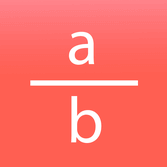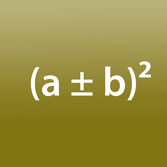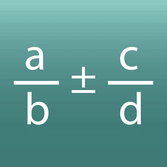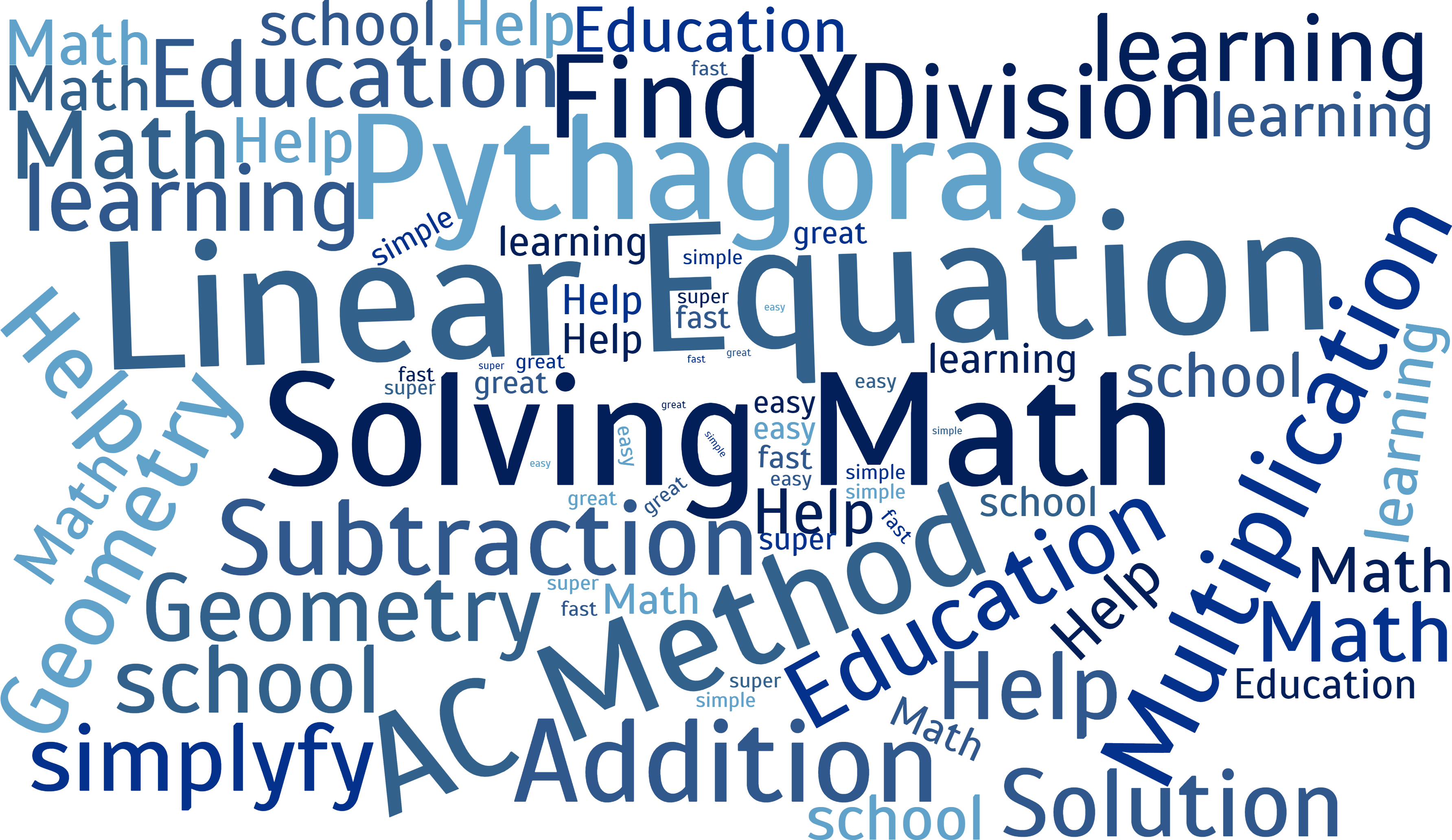# Math education with explanation and solutions

This is an educational platform for learning math with mobile solutions. Here we explain you various mathematical topics (mainly for school teaching) with texts and videos.

For each topic there is a calculator that calculates and explains the solution of a problem step by step.

Click on the black tiles below to get to the different calculators.

For each topic there is a calculator that calculates and explains the solution of a problem step by step.

Click on the black tiles below to get to the different calculators.

## Start your calculation with our solvers

Linear Equation
y = m x + nPythagoras
a² + b² = c²Reduce fractions
a / bSolving Binomial Theorem
(a ± b)²Simple Fraction Calculator
a / b ± c / d## New digital school

It’s our goal to provide free education in mathematics worldwide, mobile and multilingual. We constantly working to expand our offerings for web and smartphones.

For exciting insights into our work or to find out what's coming next, follow us on our social media channels or support us on Patreon!